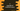# How to write a multi-line Macro in C# Introduction :

Multi-line macros are useful in many ways. For example, if you need a single if-else statement in different parts of your program, you can define a macro with the if-else condition.

In this tutorial, we will quickly learn how to write a multi-line macro in C with different examples.

## Write a multiline macro function :

Multi-line macros are similar to single line macros. The only difference is that you need to add one \ at the end of each line.

## Example 1(print the arguments) :

``````#include <stdio.h>

#define PRINT(first, second){\
printf("First : %d ", first);\
printf("Second : %d", second);\
printf("\n");\
}

int main()
{
PRINT(1,2);
PRINT(2,3);
}``````

It will print :

``````First : 1 Second : 2
First : 2 Second : 3``````

We can also write the above program as like below :

``````#include <stdio.h>

#define PRINT(first, second)\
printf("First : %d ", first);\
printf("Second : %d", second);\
printf("\n");\

int main()
{
PRINT(1,2);
PRINT(2,3);
}``````

## Example 2 : (for loop) :

``````#include < stdio.h>

#define LOOP(number)\
for (int i = 0; i < (number); i++){\
printf("%d\n", i);\
}\

int main()
{
LOOP(5);
}``````

You can use the above macro to print all numbers starting from 0 to the argument number using a for loop.

It prints the below output :

``````0
1
2
3
4``````

## Example 3 : (if-else) :

``````#include < stdio.h>

#define FIND_LARGER(first,second)\
if(first>second)\
printf("%d is greater than %d",first,second);\
else\
printf("%d is greater than %d",second,first);\

int main()
{
FIND_LARGER(2,3);
}``````

### Conclusion :

As shown in the above examples, you can convert a part of your program to a macro. It makes the code more readable and also reduces the program size. Try to run the examples shown above and drop one comment below if you have any questions.